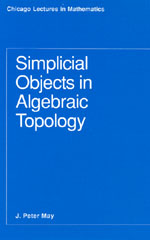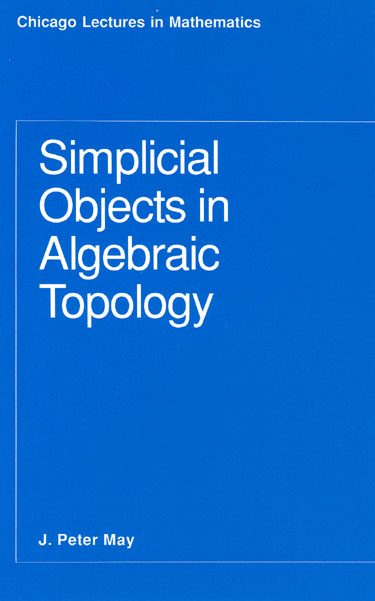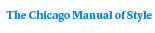# Simplicial Objects in Algebraic Topology

J. P. May170 pages | 5-1\2 x 8-1\2 | © 1967
Paper \$31.00 ISBN: 9780226511818 Published January 1993
Simplicial sets are discrete analogs of topological spaces. They have played a central role in algebraic topology ever since their introduction in the late 1940s, and they also play an important role in other areas such as geometric topology and algebraic geometry. On a formal level, the homotopy theory of simplicial sets is equivalent to the homotopy theory of topological spaces. In view of this equivalence, one can apply discrete, algebraic techniques to perform basic topological constructions. These techniques are particularly appropriate in the theory of localization and completion of topological spaces, which was developed in the early 1970s.

Since it was first published in 1967, Simplicial Objects in Algebraic Topology has been the standard reference for the theory of simplicial sets and their relationship to the homotopy theory of topological spaces. J. Peter May gives a lucid account of the basic homotopy theory of simplicial sets, together with the equivalence of homotopy theories alluded to above. The central theme is the simplicial approach to the theory of fibrations and bundles, and especially the algebraization of fibration and bundle theory in terms of "twisted Cartesian products." The Serre spectral sequence is described in terms of this algebraization. Other topics treated in detail include Eilenberg-MacLane complexes, Postnikov systems, simplicial groups, classifying complexes, simplicial Abelian groups, and acyclic models.

"Simplicial Objects in Algebraic Topology presents much of the elementary material of algebraic topology from the semi-simplicial viewpoint. It should prove very valuable to anyone wishing to learn semi-simplicial topology. [May] has included detailed proofs, and he has succeeded very well in the task of organizing a large body of previously scattered material."—Mathematical Review

Table of Contents• Contents
Contents
I. SIMPLICIAL OBJECTS AND HOMOTOPY
1. Definitions  and  examples
2. Simplicial objects in categories; homology
3. Homotopy of Kan complexes
4. The group structures
5. Homotopy of simplicial maps
6. Function complexes
Bibliographical notes on chapter I
II. FIBRATIONS, POSTNIKOV SYSTEMS, AND MINIMAL COMPLEXES
7. Kan  fibrations
8. Postnikov systems
9. Minimal complexes
10. Minimal fibrations
11. Fibre products and fibre bundles
12. Weak homotopy type
13. The Hurewicz  theorems
Bibliographical notes on chapter II
III. GEOMETRIC REALIZATION
14. The realization
16. Comparison of simplicial sets and topological spaces
Bibliographical notes on chapter III
IV. TWISTED CARTESIAN PRODUCTS AND FIBRE BUNDLES
17. Simplicial groups
18. Principal fibrations and twisted Cartesian products
19. The  group  of a  fibre  bundle
20. Fibre bundles and twisted Cartesian products
21. Universal bundles and classifying complexes
Bibliographical notes on chapter IV
V. EILENBERG-MACLANE COMPLEXES AND POSTNIKOV  SYSTEMS
22. Simplicial Abelian  groups
23. Eilenberg-MacLane complexes
24. K(rr, n)’s and cohomology operations
25. The k-invariants of Postnikov systems
Bibliographical notes on chapter V
VI. LOOP GROUPS, ACYCLIC MODELS, AND TWISTED TENSOR PRODUCTS
26.  Loop  groups
27. The  functors G, W, and  E
28.  Acyclic  models
29. The Eilenberg-Zilber theorem
30. Cup, Pontryagin, and cap products; twisting cochains
31. Brown’s  theorem
32. The Serre  spectral sequence
Bibliographical notes on chapter VI
BIBLIOGRAPHY